# K-Nearest Neighbor in Machine Learning

1. Introduction:
• Lazy learning algorithm − KNN is a lazy learning algorithm because it does not explicitly learn the model, but it saves all train data as it is in the training phase and at the testing phase, it uses whole train data for prediction. This means the training process is very fast, but the real concern is with large memory consumption to save all training data. And time complexity at testing phase because it requires to run through all train data
• Non-parametric learning algorithm − KNN is also a non-parametric learning algorithm because it doesn’t assume anything about the underlying data
• 3.1 − Calculate the distance between test data and each row of training data with the help of the Euclidean, Manhattan, Minkowski, or Hamming distance. The most commonly used method to calculate distance is Euclidean
• 3.2 − Now, based on the distance value, sort them in ascending order
• 3.3 − Next, it will select the top K rows from the sorted array
• 3.4 − Now, based on the type of model-Regression/Classification, follow the below next step:
• 3.4.1 — In the case of Classification, assign a class to the test point which is equal to the majority vote or most frequent class of the K-selected rows. If K is even, then majority votes can result in ties, it is advised to avoid even value for K
• 3.4.2 — In the case of Regression, assign a value to the test point which is equal to the mean of the K-selected rows.
• Euclidean distance
• Manhattan distance
• Minkowski Distance
• Hamming Distance
1. It is recommended to choose an odd number for K if the number of classes is 2 and another simple approach to select K=sqrt(n) where n = number of data points in the training dataset.
2. Domain knowledge is very useful in deciding the K value.
3. Using error curves: The figure below shows error curves for different values of K for training and test data. At low K values, there is overfitting of data/high variance. Therefore, test error is high, and train error is low. At K=1 in train data, the error is always zero, because the nearest neighbor to that point is that point itself. Therefore, through training error is low, test error is high at lower K values. This is called overfitting. As we increase the value for K, the test error is reduced. But after a certain K value, bias/underfitting is introduced and test error goes high. So we can say initially test data error is high(due to variance) then it goes low and stabilizes and with further increase in K value, it again increases(due to bias). The K value when test error stabilizes and is low is considered as optimal value for K. From the above error curve, we can choose K=8 for our KNN algorithm implementation.
1. Data Scaling: To locate the data point in multidimensional feature space, it would be helpful if all features are on the same scale. Hence, normalization or standardization of data will help
2. Dimensionality Reduction: KNN may not work well if there are too many features. Hence, dimensionality reduction techniques like feature selection, principal component analysis can be implemented
3. Missing value treatment: If out of M features, one feature data is missing for a particular example in the training set, then we cannot locate or calculate distance from that point. Therefore, deleting that row or imputation is required
• This algorithm is very easy to understand and interpret
• It is very useful for nonlinear data because there is no assumption about data in this algorithm
• It is a versatile algorithm, as we can use it for classification as well as regression
• Furthermore, it has relatively high accuracy, but there are much better-supervised learning models than KNN
• It is computationally a bit expensive algorithm because it stores all the training data
• High memory storage is required as compared to other supervised learning algorithms.
• Prediction is slow in the case of large data
• It is very sensitive to the scale of data as well as irrelevant features and outliers
• Banking System: KNN can be used in banking systems to predict whether an individual is fit for loan approval. Does that individual have characteristics similar to the defaulter's one?
• Calculating Credit Ratings: KNN algorithms can be used to find an individual’s credit rating by comparing it with the persons having similar traits
• Politics: With the help of KNN algorithms, we can classify a potential voter into various classes like “Will Vote”, “Will not vote”, “Will Vote to Party A”, “Will Vote to Party B”

--

--

--

## More from Heena Sharma

Love podcasts or audiobooks? Learn on the go with our new app.

## The Last Machine & Deep-Learning Compendium You’ll Ever Need.## Self Driving Car Behavioral Cloning Experiments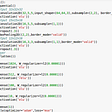## Introducing Predibase: the enterprise declarative machine learning platform## How does the Decision Tree Algorithm work?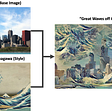## What are Convolutional Neural Networks and why are they important?## The working of RNN’s (the box that generates outputs that you receive from voice assistants like…## RAdam: A New State-of-the-Art Optimizer for RL?## Activation Function in Neural Network and Significance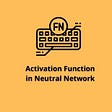## Cross-Validation Using K-Fold With Scikit-Learn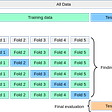## All About Decision Trees In Data Mining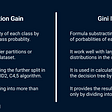## Recursive Linear Regression